# Second-order mixed partial derivative

(diff) ← Older revision | Latest revision (diff) | Newer revision → (diff)

## Definition

### For a function of two variables

Suppose$f$ is a function of two variables which we denote$x$ and$y$. There are two possible second-order mixed partial derivative functions for$f$, namely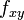$f_{xy}$ and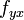$f_{yx}$. In most ordinary situations, these are equal by Clairaut's theorem on equality of mixed partials. Technically, however, they are defined somewhat differently.

Often the term mixed partial is used as shorthand for the second-order mixed partial derivative. However, mixed partial may also refer more generally to a higher partial derivative that involves differentiation with respect to multiple variables.

The following are all multiple equivalent notations and definitions of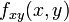$f_{xy}(x,y)$.

Name Notation Definition in terms of first-order partial derivatives
Subscript notation$f_{xy}(x,y)$ Defined as$(f_x)_y(x,y)$. More explicitly:
Let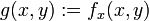$g(x,y) := f_x(x,y)$. Then,$f_{xy}(x,y) := g_y(x,y)$.
Leibniz notation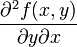$\frac{\partial^2f(x,y)}{\partial y \partial x}$ Defined as$\frac{\partial}{\partial y}\frac{\partial f(x,y)}{\partial x}$

Note that the order in which we write the$x$ and$y$ is different in the subscript and Leibniz notations because in the subscript notation, the differentiations are carried out from left to right (on subscripts) whereas in the Leibniz notation, the differentiations are carried out from right to left while simplifying.

The following are all multiple equivalent notations and definitions of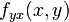$f_{yx}(x,y)$.

Notation Definition in terms of first-order partial derivatives$f_{yx}(x,y)$ Defined as$(f_y)_x(x,y)$. More explicitly:
Let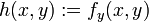$h(x,y) := f_y(x,y)$. Then,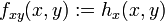$f_{xy}(x,y) := h_x(x,y)$.$\frac{\partial^2f(x,y)}{\partial x \partial y}$ Defined as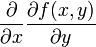$\frac{\partial}{\partial x}\frac{\partial f(x,y)}{\partial y}$

### For a function of many variables

For a function of more than two variables, we can define the second-order mixed partial derivative with respect to two of the variables (in a particular order) in the same manner as for a function of two variables, where we treat the remaining variables as constant. For instance, for a function$f$ of three variables$x,y,z$, we can consider the six mixed partials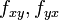$f_{xy}, f_{yx}$ (holding$z$ fixed),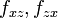$f_{xz}, f_{zx}$ (holding$y$ fixed),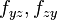$f_{yz},f_{zy}$ (holding$x$ fixed).